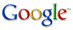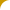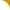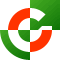Geocentrix - putting you at the centre

 Home Products Support Training Shop Forums

Scenic House, 54 Wilmot Way
+44 (0)17 3737 3963
www.geocentrix.co.uk
webmaster@geocentrix.co.uk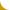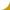# How to model non-circular pile sections in Repute 1.x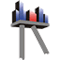Repute 1's calculation engine is designed to analyse piles with circular cross-sections. However, piles with other cross-sections can be simulated by idealising them as solid circular piles with equivalent:

• Cross-sectional area (A)
• Axial rigidity (EA)
• Bending rigidity (EI)

## Cross-sectional area

The pile's internal diameter should be set to zero and its external diameter chosen so that the pile's cross-sectional area matches that of the non-circular pile:

πDext2/4 = Aactual

» Dint = 0 and Dext = √(4Aactual / π) ...[eq'n 1]

## Axial rigidity

The pile's axial Young's modulus should be chosen so that its axial rigidity matches that of the non-circular pile:

EaxialA = EaxialπDext2/4 = (EA)actual

» Eaxial = 4(EA)actual / πDext2 ...[eq'n 2]

## Bending rigidity

The pile's lateral Young's modulus should be chosen so that its bending rigidity matches that of the non-circular pile:

ElateralI = ElateralπDext4/64 = (EI)actual

» Elateral = 64(EI)actual / πDext4 ...[eq'n 3]

## Example 1 - square pile

Consider a 0.6m x 0.6m square section pile (A = B2, I = B4/12) made out of concrete (E = 35 GPa):

Aactual = 0.6 x 0.6 = 0.36 m2 = 3600 cm2
(EA)actual = 35 x 0.36 = 12.6 GN = 12600 MN
(EI)actual = 35 x 0.64/12 = 378 MNm2

The equivalent circular pile to enter into Repute is:

Dint = 0
Dext = √(4 x 0.36 / π) = 0.677 m
Eaxial = (4 x 12.6) / x 0.6772) = 35.0 GPa
Elateral = (64 x 378) / (π x 0.6774) = 36.47 GPa

## Example 2 - H-section pile

Consider a 254 x 254 x 63 universal (H-section) bearing pile (A = 79.7 cm2; Ixx = 8775 cm4) made out of steel (E = 200 GPa).

The relevant cross-sectional area for equation  is the gross enclosed area of the pile (Agross = B2), but for equation , based on axial rigidity, it is the actual cross-sectional area of the pile (Aactual = 79.7 cm2), so:

Agross = 0.254 x 0.254 = 0.0645 m2
(EA)actual = 200 x 0.00797 = 1.59 GN
(EI)actual = 200 x 0.00008775 = 17.55 MNm2

The equivalent circular pile to enter into Repute is:

Dint = 0
Dext = √(4 x 0.0645 / π) = 0.287 m
Eaxial = (4 x 1.59) / (π x 0.2872) = 24.6 GPa
Elateral = (64 x 17.55) / (π x 0.2874) = 52.7 GPaOnline shop
Contact details
How to find us

### Products

Geocentrix ReActiv
Geocentrix Redoubt
Geocentrix Registrar
Geocentrix Renown
Geocentrix Repute
Geocentrix ReWaRD
Special offers

### Training courses

Decoding Eurocodes 2+3+7
ReWaRD Hands-on
Repute 2 Hands-on